• ## 用python绘制散点图

万次阅读 多人点赞 2018-07-13 21:41:53
今天下午学习了如何使用python绘制简单的散点图，写成博客分享一下。 在python中画散点图主要是用matplotlib模块中的scatter函数，先来看一下scatter函数的基本信息。 网址为：点击打开链接 可以看到scatter中有很多...
在python中画散点图主要是用matplotlib模块中的scatter函数，先来看一下scatter函数的基本信息。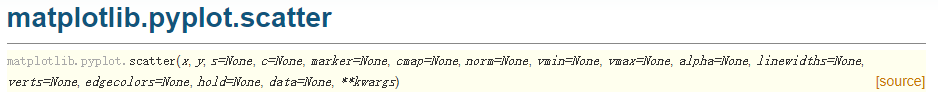网址为：点击打开链接
可以看到scatter中有很多参数，经常使用的参数主要有以下几个：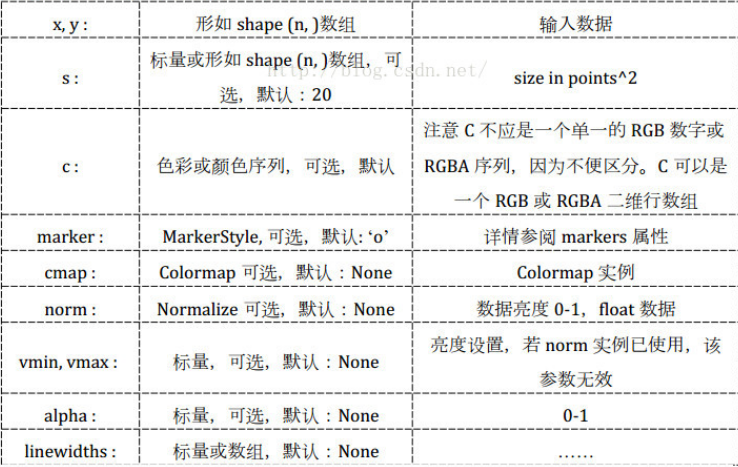c：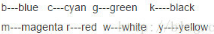marker: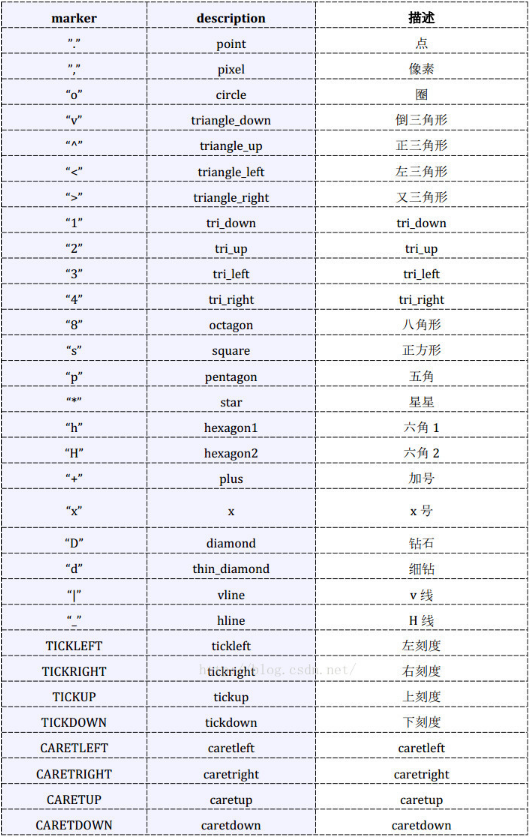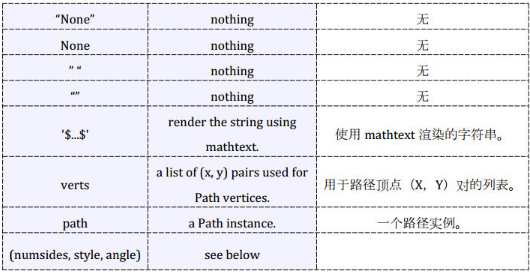数据、代码和绘制的图如下。
数据（取第一列作为x，取第四列作为y）截图：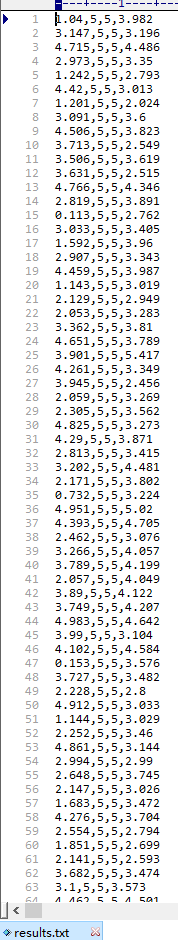代码如下：
import matplotlib.pyplot as plt
import numpy as np

# 定义画散点图的函数
def draw_scatter(n, s):
"""
:param n: 点的数量，整数
:param s:点的大小，整数
:return: None
"""
# 加载数据
# 通过切片获取横坐标x1
x1 = data[:, 0]
# 通过切片获取纵坐标R
y1 = data[:, 3]
# 横坐标x2
x2 = np.random.uniform(0, 5, n)
# 纵坐标y2
y2 = np.array( * n)
# 创建画图窗口
fig = plt.figure()
# 将画图窗口分成1行1列，选择第一块区域作子图
# 设置标题
ax1.set_title('Result Analysis')
# 设置横坐标名称
ax1.set_xlabel('gamma-value')
# 设置纵坐标名称
ax1.set_ylabel('R-value')
# 画散点图
ax1.scatter(x1, y1, s=s, c='k', marker='.')
# 画直线图
ax1.plot(x2, y2, c='b', ls='--')
# 调整横坐标的上下界
plt.xlim(xmax=5, xmin=0)
# 显示
plt.show()

# 主模块
if __name__ == "__main__":
# 运行
draw_scatter(n=2000, s=20)
绘制的图如下：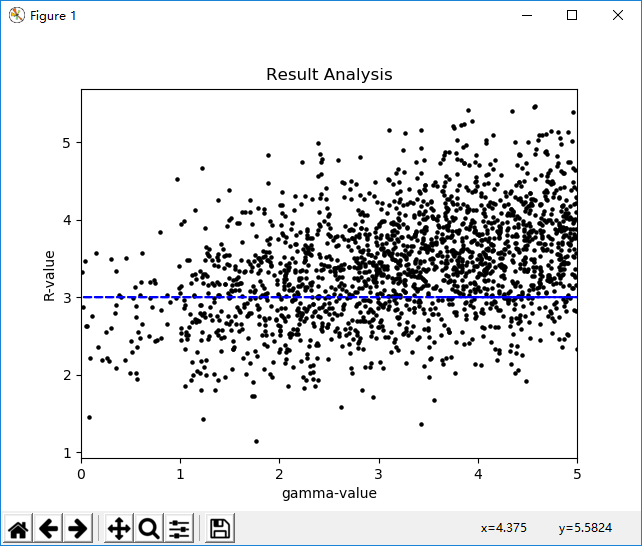展开全文scatter
• python散点图 Python-散点图 (Python - Scatter Plots) Advertisements 广告 Previous Page 上一页 Next Page 下一页 Scatterplots show many points plotted in the Cartesian plane. Each point ...python散点图
Python-散点图 (Python - Scatter Plots)
Scatterplots show many points plotted in the Cartesian plane. Each point represents the values of two variables. One variable is chosen in the horizontal axis and another in the vertical axis.
散点图显示在笛卡尔平面中绘制的许多点。 每个点代表两个变量的值。 在水平轴上选择一个变量，在垂直轴上选择另一个变量。
绘制散点图 (Drawing a Scatter Plot)
Scatter plot can be created using the DataFrame.plot.scatter() methods.
可以使用DataFrame.plot.scatter()方法创建散点图。

import pandas as pd
import numpy as np
df = pd.DataFrame(np.random.rand(50, 4), columns=['a', 'b', 'c', 'd'])
df.plot.scatter(x='a', y='b')


Its output is as follows −
其输出如下-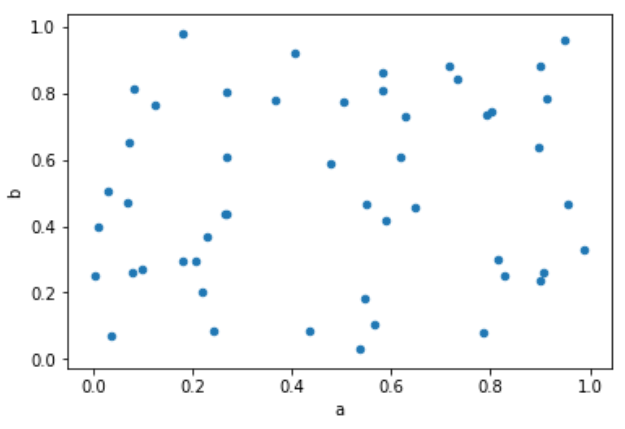翻译自: https://www.tutorialspoint.com/python_data_science/python_scatter_plots.htm

python散点图

展开全文• 散点图可以直观的查看数据的分布情况。 matplotlib模块的pyplot有画散点图的函数，但是该函数要求x轴是数字类型。 pandas的plot函数里，散点图类型scatter也要求数字型的，用时间类型的会报错。 在搜索阅读了几十...广告关闭
腾讯云11.11云上盛惠 ，精选热门产品助力上云，云服务器首年88元起，买的越多返的越多，最高返5000元！用散点图可以直观的查看数据的分布情况。 matplotlib模块的pyplot有画散点图的函数，但是该函数要求x轴是数字类型。 pandas的plot函数里，散点图类型scatter也要求数字型的，用时间类型的会报错。 在搜索阅读了几十篇网文后，摸索出画散点图的简单办法。 可以使用pyplot的plot_date()画散点图。 下面是完整的python...
我现在有一个nx3矩阵数组。 我想把这三列画成三轴，我怎么能这样做呢？ 我在谷歌上搜索过，人们建议用matlab但是我真的很难理解它。 我还需要一个散点图。 有人能教我吗？...
关联图是查找两个事物之间关系的图像，他能为我们展示出一个事物随着另一个事物的变化如何变化。 典型的关联图有：折线图、散点图、相关矩阵等我们什么时候会需要关联图？ 1、数据报告 & 学术研究展示趋势：比如产品销量随着时间如何变化，智力水平随着教育程度如何变化等展现状态：不同年龄的客户的成交率...下面是一个制作散点图动画的例子：import plotly.express as pxdf = px.data.gapminder()fig =px.scatter( df, x=gdppercap, y=lifeexp, animation_frame=...而漂漂亮亮的可视化是完成这一任务的绝佳工具。 本文将介绍 5 种非传统的可视化技术，可让你的数据故事更漂亮和更有效。 这里将使用 python 的 plotly ...
下面是一个制作散点图动画的例子：import plotly.express as pxdf = px.data.gapminder()fig =px.scatter( df, x=gdppercap, y=lifeexp, animation_frame=...而漂漂亮亮的可视化是完成这一任务的绝佳工具。 本文将介绍 5 种非传统的可视化技术，可让你的数据故事更漂亮和更有效。 这里将使用 python 的 plotly ...来自维基百科不过，我们仍然可以绘制出多维空间，今天就来用 python 的 plotly 库绘制下三维到六维的图，看看长什么样。 数据我们使用一份来自 uci 的真实汽车数据集，该数据集包括 205 个样本和 26 个特征，从中选择 6 个特征来绘制图形：? 基础工作安装好 plotly 包：pip install plotly加载数据集（文末会提供）...
今日锦囊常用的统计图在python里怎么画? 这里的话我们介绍几种很简单但也很实用的统计图绘制方法，分别有条形图、饼图、箱体图、直方图以及散点图，关于这几种图形的含义这边就不多做解释了。 今天用到两个数据集，数据集大家可以在公众号回复特征工程来获取，分别是salary_ranges_by_job_classification和globalland...下面，我们使用python画一些plolty基本图的demo：（大家如果有需要用plotly的建议收藏哦！ 散点图散点图核心的价值在于发现变量之间的关系，千万不要简单地将这个关系理解为线性回归关系。 变量间的关系有很多，如线性关系、指数关系、对数关系等等，当然，没有关系也是一种重要的关系。 散点图更偏向于研究型图表...
选自towardsdatascience作者：william koehrsen机器之心编译参与：nurhachu null、路 本文介绍了如何在 python中利用散点图矩阵（pairs plots）进行数据可视化。 如何快速构建强大的探索性数据分析可视化当你得到一个很不错的干净数据集时，下一步就是探索性数据分析（exploratory data analysis，eda）。 eda 可以...与线型图类似的是，散点图也是一个个点集构成的。 但不同之处在于，散点图的各点之间不会按照前后关系以线条连接起来。 用plt.plot画散点图? 奇怪，代码和前面的例子差不多，为什么这里显示的却是散点图而不是sin曲线呢？ 原因有二：一是点集比较少，稀疏，才30个； 二是没有指定线型。 用plt.scatter画散点图scatter...
使用matplotlib画散点图步骤，第一种使用scatter(x,y),系统自动建立坐标系，第二种使用plot(x,y)系统也是自动建立坐标系，plot函数默认画连线图。 比较，scatter比plot适合画散点图。 7、 pandas是一个为解决python数据分析而用的包，可以快速构建数据结构。 8、scikit-learn简称sklearn,在导入数据包时只能使用...下面是一个制作散点图动画的例子：import plotly.express as pxdf = px.data.gapminder()fig =px.scatter( df, x=gdppercap, y=lifeexp, animation_frame=...而漂漂亮亮的可视化是完成这一任务的绝佳工具。 本文将介绍 5 种非传统的可视化技术，可让你的数据故事更漂亮和更有效。 这里将使用 python 的 plotly ...画散点图：# -*- coding:utf-8 -*-import pandas as pdimport numpy as npfrom sklearn.decompositionimport pcafrom sklearn.linear_model import logist...plt.grid(true)##画出虚线方格 plt.show()? 我们可以绘制在三维空间的正态分布图代码如下#! usrbinpython# -*- coding:utf-8 -*-import numpy as npfrom ...案件回顾饭团销售额下滑现有冰激凌店一年的历史销售数据数据包括单日的销售量、气温、周几（问题：如何用这些数据预测冰激凌的销量？ 模拟实验与分析将数据存储为csv格式，导入python。 并画出散点图，观察气温和销售量的关系。 import pandas as pdicecream = pd.read_csv(icecream.csv)importmatplotlib.pyplot as ...
一般分三步：（1）画散点图，找模型； （2）进行回归模型的参数估计； （3）检验前面分析得到的经验模型是否合适。 画散点图创建一个datatemp的文件夹...上一篇文章讲了最小二乘算法的原理。 这篇文章通过一个简单的例子来看如何通过python实现最小乘法的线性回归模型的参数估计。 王松桂老师《线性统计模型—...
2.pngdef print_scatter_draw(): 画散点图 return:x=np.random.rand(1000) y=np.random.rand(len(x)) #绘图plt.scatter(x,y,color=b,label=scatter draw, ...5.pngcopy的文章 数据挖掘之matplotlib入门发现python的代码粘贴过去，因为缩进，需要重新修改，有点麻烦 百度云 源码分享，下载下来，体验运行吧。? 图像...datinglabels = file2matrix(datingtestset2.txt) import matplotlibimportmatplotlib.pyplot as pltfig = plt.figure()ax =fig.add_subplot(111)ax.scatter(datingdatamat, datingdatamat, 15.0*array(datinglabels),15.0*array(datinglabels)) #scatter函数是用来画散点图的plt.show() 结果显示 1-4. 准备数据： ...datinglabels = file2matrix(datingtestset2.txt) 1 import matplotlib2 importmatplotlib.pyplot as plt3 fig = plt.figure()4 ax = fig.add_subplot(111)5ax.scatter(datingdatamat, datingdatamat, 15.0*array(datinglabels),15.0*array(datinglabels)) #scatter函数是用来画散点图的6 plt.show()结果显示?...
流程图如下：扩容python 会根据散列表的拥挤程度扩容。 “扩容”指的是:创造更大的数组，将原有内容拷贝到新数组中。 接近 23 时，数组就会扩容。 根据键查找“键值对”的底层过程我们明白了，一个键值对是如何存储到数组中的，根据键对象取到值对象，理解起来就简单了。 a.get(name)gaoqi当我们调用 a.get(“name”)...
画散点图如下：# 散点图from pylab import *n = 1024x = np.random.normal(0,1,n)y =np.random.normal(0,1,n)scatter(x,y)show()显示： ? 画条形图如下：#条形图from pylab import *n = 12x = np.arange(n)y1 = (1-xfloat(n)) *np.random.uniform(0.5,1.0,n)y2 = (1-xfloat(n)) *np.random.uniform(0.5,1.0,n)bar(x, ...
展开全文• 环境：windows系统，anaconda3 64位，python 3.61.初认识基本代码如下：import numpy as npimport matplotlib.pyplot as pltN = 1000x = np.random.randn(N)y = np.random.randn(N)plt.scatter(x, y)plt.show()这里...
环境：windows系统，anaconda3 64位，python 3.6
1.初认识
基本代码如下：
import numpy as np
import matplotlib.pyplot as plt
N = 1000
x = np.random.randn(N)
y = np.random.randn(N)
plt.scatter(x, y)
plt.show()
这里使用numpy包的random函数随机生成1000组数据，然后通过scatter函数绘制了散点图。随机散点图
这篇文章的重点其实在于scatter函数。scatter
x,y 形如shape(n,)的数组，可选值，
s 点的大小（也就是面积）默认20
c 点的颜色或颜色序列，默认蓝色。其它如c = 'r' (red); c = 'g' (green); c = 'k' (black) ; c = 'y'(yellow)
marker 形状，可选值，默认是圆基本
如果需要其他的，可搜索matplotlib的官网，在官网中搜索markers，选择第一个结果。
import numpy as np
import matplotlib.pyplot as plt
N = 1000
x = np.random.randn(N)
y = np.random.randn(N)
color = ['r','y','k','g','m']
plt.scatter(x, y,c=color,marker='>')
plt.show()示例
alpha：标量，可选，默认值：无， 0（透明）和1（不透明）之间的alpha混合值
import numpy as np
import matplotlib.pyplot as plt
N = 1000
x = np.random.randn(N)
y = np.random.randn(N)
plt.scatter(x, y,alpha=0.5)
plt.show()示例alpha = 0.5
edgecolors，顾名思义，边缘颜色或颜色序列，可选值，默认值：None
import numpy as np
import matplotlib.pyplot as plt
N = 1000
x = np.random.randn(N)
y = np.random.randn(N)
plt.scatter(x, y,alpha=0.5,edgecolors= 'white') #edgecolors = 'w',亦可
plt.show()与上图对比
2.图例无法显示中文
import numpy as np
import matplotlib.pyplot as plt
N = 1000
x = np.random.randn(N)
y = np.random.randn(N)
plt.scatter(x, y,alpha=0.5,edgecolors= 'white')
plt.title('示例')#显示图表标题
plt.xlabel('x轴')#x轴名称
plt.ylabel('y轴')#y轴名称
plt.grid(True)#显示网格线
plt.show()中文无法显示
查找原因，发现时因为matplotlib库没有中文字体。
解决方案1
每次编代码时都进行参数设置如下：
#coding:utf-8
import matplotlib.pyplot as plt
plt.rcParams['font.sans-serif']=['SimHei'] #用来正常显示中文标签
plt.rcParams['axes.unicode_minus']=False #用来正常显示负号
#有中文出现的情况，需要u'内容'
解决方案2
说实话，我折腾了半天没有成功，只有临时方法成功了。
等下次在尝试。
待续
展开全文• 环境：windows系统，anaconda3 64位，python 3.61.初认识基本代码如下：import numpy as npimport matplotlib.pyplot as pltN = 1000x = np.random.randn(N)y = np.random.randn(N)plt.scatter(x, y)plt.show()这里...
• 本代码是利用python绘制散点图，使用xlrd读取Excel文件，
• python绘制散点图标签：#Python##散点图#时间：2019/03/27 21:13:00作者：夏天的风今天下午学习了如何使用python绘制简单的散点图，写成博客分享一下。在python中画散点图主要是用matplotlib模块中的scatter函数，...
• 其在Python中得API接口包名为folium（不知道包作者为何这样起名字，和leaflet已经扯不上关系了），可以满足我们平时常用的热力、填充地图、路径散点标记等高频可视化场景。关于folium在热力上的用法，可以...
• 散点图和趋势线的简单介绍： 散点图一般用于描述两个数量型变量之间的相关关系，而趋势线是显示相关性近似程度的一条直线。 绘制散点图的主要方法： 方法1：通过pandas包中的DataFrame对象调用plot()或plot....数据分析
• python散点图问题描述实例数据准备绘制散点图结果展示参考 问题描述 总结一个普遍的画图格式太难了，我就举一个比较复杂的例子来说明，比如我有一个嵌入向量矩阵(N*128)，想将其可视化到二维平面，所以就用t-sne将...可视化 pandas
• ## python_散点图矩阵图

千次阅读 2019-12-05 14:15:09
python_散点图矩阵图 使用散点图矩阵图，可以两两发现特征之间的联系 参考此链接： https://blog.csdn.net/wangxingfan316/article/details/80033557
• 与线型图类似的是，散点图也是一个个点集构成的。但不同之处在于，散点图的各点之间不会按照前后关系以线条连接起来。用plt.plot画散点图奇怪，代码和前面的例子差不多，为什么这里显示的却是散点图而不是sin曲线呢...
• 比如五角星的散点图： 圆点的散点图： Y形的散点图： 等等还有很多，那么这些漂亮的形状是怎么画出来的呢？其实代码很简单： from numpy import *; N = 50 # 点的个数 x = np.random.rand(N) * 2 # 随机产生50个0~2...
• 今天小编就为大家分享一篇python绘制散点图并标记序号的方法，具有很好的参考价值，希望对大家有所帮助。一起跟随小编过来看看吧
• 前言本文档旨在记录通过python的matplotlib包绘制散点图的实现细节需求分析1.将excel的数据导入python2.通过python绘制散点图1）不同类别的点标注不同的颜色；2）绘制图例；具体实现需求1：将excel的数据导入python...
• 散点图可以直观的查看数据的分布情况。matplotlib模块的pyplot有画散点图的函数，但是该函数要求x轴是数字类型。pandas的plot函数里，散点图类型'scatter'也要求数字型的，用时间类型的会报错。在搜索阅读了几十篇...
• 主要为大家详细介绍了python scatter散点图用循环分类法加图例，具有一定的参考价值，感兴趣的小伙伴们可以参考一下
• matplotlib模块的pyplot有画散点图的函数，但是该函数要求x轴是数字类型。pandas的plot函数里，散点图类型"scatter"也要求数字型的，用时间类型的会报错。在搜索阅读了几十篇网文后，摸索出画散点图的简单...
• python 调用opencv 实现图像的导入和显示 应该对于想学习python初学者有点用
• I am using Python matplotlib. i want to superimpose scatter plots. I know how to superimpose continuous line plots with commands:>>> plt.plot(seriesX)>>> plt.plot(Xresampl)>>> plt.show()But it does no...
• ## 用 python 画散点图与气泡图

万次阅读 多人点赞 2019-08-23 00:27:15
散点图是数据点在直角坐标系平面上的分布图，在统计学的回归分析与预测中经常用到。用横轴代表变量 xxx，纵轴代表变量 yyy，每组数据 (xi,yi)(x_i, y_i)(xi​,yi​) 在坐标系中用一个点表示。 做散点图要用到 pyplot...气泡图
• Python散点图 # 2020.01.06 By yangbocsu 参考：https://blog.csdn.net/w576233728/article/details/86538060 import numpy as np import matplotlib.pyplot as plt #matplotlib画图中中文显示会有问题，需要...
• 散点图可以直观的查看数据的分布情况。matplotlib模块的pyplot有画散点图的函数，但是该函数要求x轴是数字类型。pandas的plot函数里，散点图类型'scatter'也要求数字型的，用时间类型的会报错。在搜索阅读了几十篇...
• ## Python画散点图

千次阅读 2020-07-19 18:12:42
import matplotlib.pyplot as plt import numpy as np import pandas as pd my_dpi=96 plt.figure(figsize=(480/my_dpi, 480/my_dpi), dpi=my_dpi) df=pd.DataFrame({'x': range(1,101), 'y': np.random.randn(100...
• 比如五角星的散点图： 圆点的散点图： Y形的散点图： 等等还有很多，那么这些漂亮的形状是怎么画出来的呢？其实代码很简单： from numpy import *; N = 50 # 点的个数 x = np.random.rand(N) * 2 # 随机产生50个0~2......

# python作散点图python 订阅